## Example Questions

1 2 4 Next →

### Example Question #5 : Completing Incomplete Tables

A middle school teacher conducted a survey of the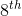grade class and found that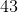students were athletes andof those students drink soda. There werestudents that were not athletes but drank soda. Last, they found thatstudents did not have a curfew nor were on the honor roll. Given this information, how many students drink soda?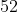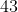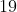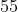Explanation:

To help answer this question, we can construct a two-way table and fill in our known quantities from the question.

The columns of the table will represent the students who are athletes or are not athletes and the rows will contain the students who drink soda or do not drink soda. The first bit of information that we were given from the question was thatstudents were athletes; therefore,needs to go in the "athlete" column as the row total. Next, we were told that of those students,drinks soda; therefore, we need to putin the "athlete" column and in the "drinks soda" row. Then, we were told thatstudents were not athletes, but drink soda, so we need to putin the "not an athlete" column and the "drinks soda" row. Finally, we were told thatstudents are not athletes or soda drinkers, soneeds to go in the "not an athlete" column and "doesn't drink soda" row. If done correctly, you should create a table similar to the following: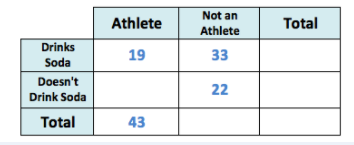Our question asked how many students drink soda. We add up the numbers in the "drinks soda" row to get the total: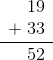This means thatstudents drink soda.

### Example Question #10 : Completing Incomplete Tables

A middle school teacher conducted a survey of thegrade class and found thatstudents were athletes andof those students drink soda. There werestudents that were not athletes, but drank soda. Last, they found thatstudents were neither athletes nor drank soda. Given this information, how many students don't drink soda?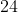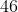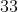Explanation:

To help answer this question, we can construct a two-way table and fill in our known quantities from the question.

The columns of the table will represent the students who are athletes or are not athletes and the rows will contain the students who drink soda or do not drink soda. The first bit of information that we were given from the question was thatstudents were athletes; therefore,needs to go in the "athlete" column as the row total. Next, we were told that of those students,drinks soda; therefore, we need to putin the "athlete" column and in the "drinks soda" row. Then, we were told thatstudents were not athletes, but drink soda, so we need to putin the "not an athlete" column and the "drinks soda" row. Finally, we were told thatstudents are not athletes or soda drinkers, soneeds to go in the "not an athlete" column and "doesn't drink soda" row. If done correctly, you should create a table similar to the following: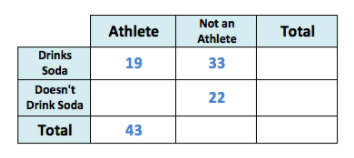Our question asked how many students don't drink soda. We add up the numbers in the "doesn't drink soda" row to get the total, but first, we need to fill in a gap in our table, students who were athletes, but don't drink soda. We can take the total number of students who are athletes,, and subtract the number of students who drink soda,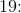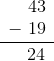This means thatstudents who are athletes, don't drink soda.

Now, we add up the numbers in the "doesn't drink soda" row to get the total: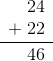This means thatstudents don't drink soda.

1 2 4 Next →

### All SAT Mathematics Resources